Electricity & Megnetism

# Electrical potential energy

Electrical potential energy is a form of energy related to the relative position between pairs of electrical charges . Electrical potential energy is a scalar quantity , measured in joules, which can be calculated by multiplying the magnitude of the test charge, measured in coulombs, by the electrical potential , in volts.

## Definition of electrical potential energy

Every electric charge produces an electric field that permeates through space, being able to produce forces of attraction or repulsion on other electric charges. The interaction between charges, therefore, gives rise to a potential energy, which can be transformed into kinetic energy , in the case where one of these charges is mobile, for example.

By itself, an electric charge does not present electric potential energy, it is necessary that a test charge interacts with it, in this way, the measure of the electric potential energy depends on the magnitude of the charges , as well as the distance between them.

Electric potential energy is a scalar quantity, and therefore, to know the measure of the total electric potential energy of a system of several charged bodies, it is necessary to add the potential energy generated by the interaction of each pair of electrified bodies .

## Electric potential energy formula

The formula for electric potential energy (E P ) is given by the product between the generating electric charge (Q), the test electric charge ( q), and the electrostatic vacuum constant (k 0 ), divided by the distance between the charges, as shown below: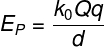In addition to this formula, it is possible to calculate the electrical potential energy through the electrical potential produced by the generating load (U) multiplied by the magnitude of the test electrical charge. Taking into account the sign of electrical potential energy, it is understood that a negative electrical potential energy is related to the attraction between charges, while a positive sign refers to the repulsion between charges.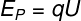## Example of electrical potential energy

Here’s an example of how to use the electric potential energy formula:

Two electric charges of 2.0 μC and -3.0 μC are fixed and separated by a distance of 0.5 m in vacuum. Calculate the electrical potential energy generated by the interaction between these charges.

Resolution :

To solve the example, we must remember the unit prefixes , very common for the denotation of electrical charges, which usually require very small units of measurement . Then, knowing that the prefix μ is equivalent to 10 -6 , we must develop the calculation of electric potential energy: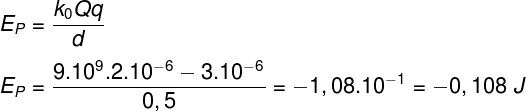## Electric potential energy in the capacitor

Capacitors are devices used for the storage of electrical charges , that said, it is possible to calculate the amount of electrical potential energy stored between the conductive armatures of the capacitors To do so, we need to know some parameters, such as the stored electrical charge , the potential difference between the plates, or even the capacitance .

The following figure shows the different formulas used to calculate the electrical potential energy in capacitors: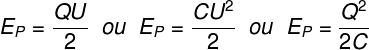Q – electrical charge

U – electric potential

C – capacitance

## Electric potential and electric potential energy

Electric potential and electric potential energy are different but related things . While electrical potential is measured in volts, electrical potential energy is measured in joules , for example. Electric potential is a property of each electric charge, while electric potential energy is a product of the interaction between pairs of charges and does not exist in solitary charges.

The relationship between these two quantities is as follows: the electric potential is equal to the electric potential energy per unit of charge, that is, the amount of volts in a certain region of space determines what the electric potential energy will be for each 1 C of charge. test electrical.

## Variation of electrical potential energy

The variation of electric potential energy occurs thanks to the performance of work by the action of the electric force . When a mobile charge is positioned in the vicinity of a fixed charge, for example, the electric force transforms the electric potential energy into kinetic energy, so we can say that the variation of the electric potential energy is equivalent to the work done, as well as the variation of the electric potential energy. kinetic energy acquired by moving charges .

The formula that relates change in electrical potential energy, work and change in kinetic energy is shown below, note: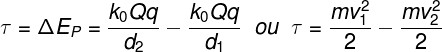2 ed 1 – positions 1 and 2 of the particle

1 and v 2 – particle velocities 1 and 2

## Exercises on electrical potential energy

Question 1) About electric potential energy, mark the correct alternative:

a) Electric potential energy is the amount of electric charge stored in an electrified body.

b) Electric potential energy is a scalar quantity, measured in joules.

c) Electric potential energy is a vector quantity, measured in volts.

d) Electric potential energy is a measure of the passage of electric charges.

e) Electric potential energy is one of the known forms of mechanical energy.

Template: letter b

Resolution :

Electric potential energy is a scalar quantity, measured in joules, its nature is electromagnetic, since it arises from the interaction between electric charges, therefore, the correct alternative is letter b.

Question 2) Determine the intensity of the electric potential energy of a system composed of two fixed charges of 1.0 mC and 0.5 mC, and 0.25 m distant from each other, and mark the corresponding alternative:

Use 0 = 9.10 9 N.m²/C²

a) 1.8.10 4 J

b) 2.7.10 4 J

c) 3.5.10 5 J

d) 0.9.10 3 J

e) 1.2.10 6 J

Template : letter a

Resolution:

Let’s use the electric potential energy formula to solve the exercise, note: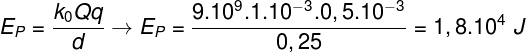According to the calculation, the electrical potential energy of this system is equal to 1.8. 10^4 J. Therefore, the correct alternative is letter a.